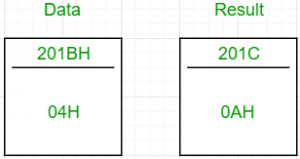# 8085 program to find the sum of first n natural numbers

Problem – Write an assembly language program for calculating the sum of first n natural numbers using 8085 microprocessor.

Example –

Input : 04H
Output : 0AH
as 01+02+03+04 = 10 in decimal => 0AHThe formula for calculating the sum of first n natural numbers is.

Algorithm –

1. With n as the input, increment it to obtain n+1.
2. Multiply n with n+1.
3. Divide the product obtained by 2.

In 8085 microprocessor, no direct instruction exists to multiply two numbers, so multiplication is done by repeated addition as 4×5 is equivalent to 4+4+4+4+4 (i.e., 5 times).
Input: 04H
Product: 14H(2010)

Similarly, in 8085 microprocessor, no direct instruction exists to divide two numbers, so division is done by repeated subtraction.
Input: 14H
Keep subtracting 2 from the input till it reduces to 0.
Since subtraction has to be performed 1010 times before 14H becomes 0, the quotient is 1010 => 0AH.

Steps –

1. Load the data from the memory location (201BH, arbitrary choice) into the accumulator
2. Move this data into B
3. Increment the value in the accumulator by one and move it to the register C
4. Initialise the accumulator with 0
5. Multiplication: Keep adding B to accumulator. The number of times B has to be added is equal to the value of C
6. Initialise B with 00H. B will store the quotient of the division
7. Initialise C with 02H. This is the divisor for the division
8. Division: Keep subtracting C from A till A becomes 0. For each subtraction, increment B by one
9. The final answer is in B. Move it to A. Then store the value of A in 201CH (arbitrary choice again)

2000H LDA 201BH
2001H
2002H
2003H MOV B, A
2004H INR A
2005H MOV C, A
2006H MVI A, 00H
2007H
2009H DCR C
200AH JNZ LOOP1
200BH
200CH
200DH MVI C, 02H
200EH
200FH MVI B, 00H
2010H
2011H LOOP2 INR B
2012H SUB C
2013H JNZ LOOP2
2014H
2015H
2016H MOV A, B
2017H STA 201CH
2018H
2019H
201AH HLT

Store the value of n in 201BH. The sum can be found at 201CH.

My Personal Notes arrow_drop_upCheck out this Author's contributed articles.

If you like GeeksforGeeks and would like to contribute, you can also write an article using contribute.geeksforgeeks.org or mail your article to contribute@geeksforgeeks.org. See your article appearing on the GeeksforGeeks main page and help other Geeks.

Please Improve this article if you find anything incorrect by clicking on the "Improve Article" button below.

Improved By : nidhi_biet